# Distribution Coefficients Lab

### Introduction

The distribution coefficient, also known as the partition coefficient, is a fundamental concept in chemistry used to predict the distribution of a solute between two immiscible phases; aqueous phase and organic phase. This experiment aims to demonstrate the principles of distribution coefficients by performing a separation of two organic acids, crotonic acid and benzoic acid, between aqueous and organic solvents using a separatory funnel. If performed correctly, we anticipate that benzoic acid will have a much higher distribution constant than crotonic acid, indicating a higher solubility in organic phases than in aqueous phases.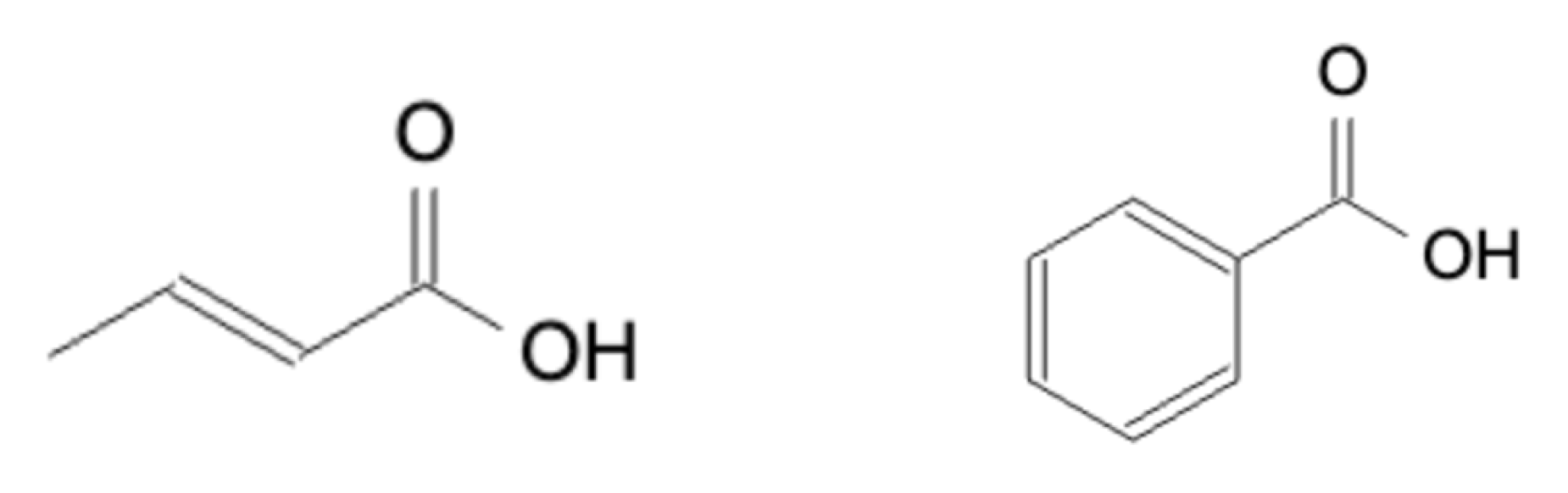Figure 1: Crotonic and Benzoic Acid

### Materials and Methods

#### Crotonic Acid

Using a graduated cylinder, 25mL of deionized water and 25mL of methylene chloride was added to a separatory funnel. 108.7mg of crotonic acid was weighed and added to the solution. The solution was mixed by capping the separatory funnel and shaking. Once mixed, the separatory funnel was allowed to stand until both layers were completely separated. The bottom layer was drawn off into a 125mL flask labeled “Flask 1” and the remaining solution in the separatory funnel was swirled around and allowed to settle again. The bottom layer was again drawn off into Flask 1 at which point, another 125mL flask labeled “Flask 2” was used to collect the remaining layer of the separatory funnel. 3mL of deionized water was added to the separatory funnel and swirled to collect the remaining aqueous layer, and then drawn into Flask 2.

Four drops of phenolphthalein indicator were added to Flask 2 and titrated with 0.10M NaOH and swirled by hand constantly until a faint, permanent pink color was achieved. This process was repeated for Flask 1.

#### Benzoic Acid

Using a graduated cylinder, 25mL of deionized water and 25mL of methylene chloride was added to a separatory funnel. 152.6mg of benzoic acid was weighed and added to the solution. The solution was mixed by capping the separatory funnel and shaking. Once mixed, the separatory funnel was allowed to stand until both layers were completely separated. The bottom layer was drawn off into a 125mL flask labeled “Organic Phase”. The top layer was collected into another 125mL flask labeled “Aqueous Phase”. 3mL of this solution was put back into the separatory funnel, swirled, and collected.

Four drops of phenolphthalein indicator were added to the “Aqueous Phase” flask and titrated with 0.10M NaOH and swirled by hand constantly until a faint, permanent pink color was achieved.

### Results

For the crotonic acid experiment, 5.72 mL and 7.28mL of 0.10M NaOH was needed to activate the phenolphthalein indicator in the organic (Flask 1) and aqueous (Flask 2) phase respectively. Using the 0.10M NaOH titration volumes, the distribution coefficient can be calculated using the equation below: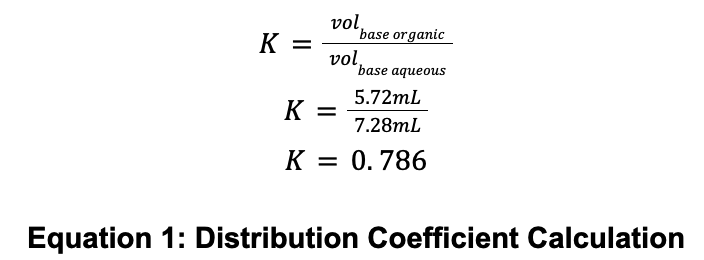Using the distribution coefficient and the starting weight of 108.7g of crotonic acid, the amount of crotonic acid in each can be estimated: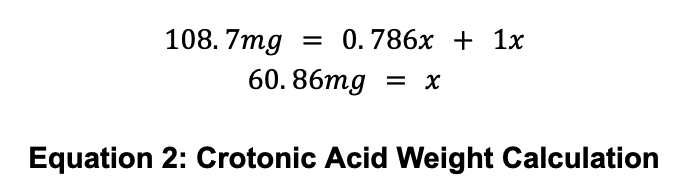For the benzoic acid experiment, 1.27mL of 0.10M NaOH was needed to activate the phenolphthalein indicator in the Aqueous Phase flask. To calculate the amount of benzoic acid in the organic phase, the weight in the organic phase must be determined and subtracted from the total weight of benzoic acid used (152.6mg):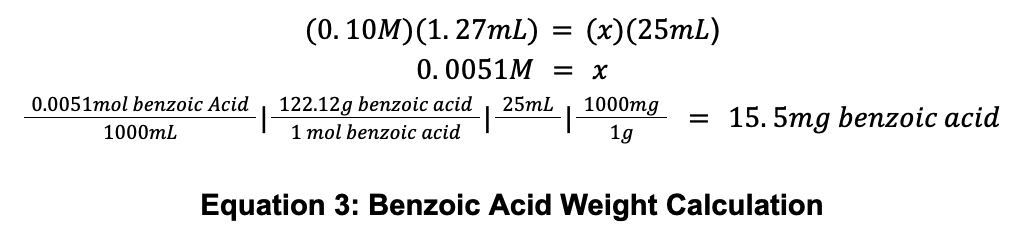The benzoic acid solution starting weight was 152.6mg, and with 15.5mg of benzoic acid in the aqueous phase, it is calculated that there is 137.1mg in the organic phase. Using these values provides a separation coefficient of 8.85:### Discussion

The results show that crotonic acid has a distribution coefficient of 0.786 and benzoic acid has a K of 8.85. When evaluating the resonance structures of benzoic acid and crotonic acid, the reason why becomes clear: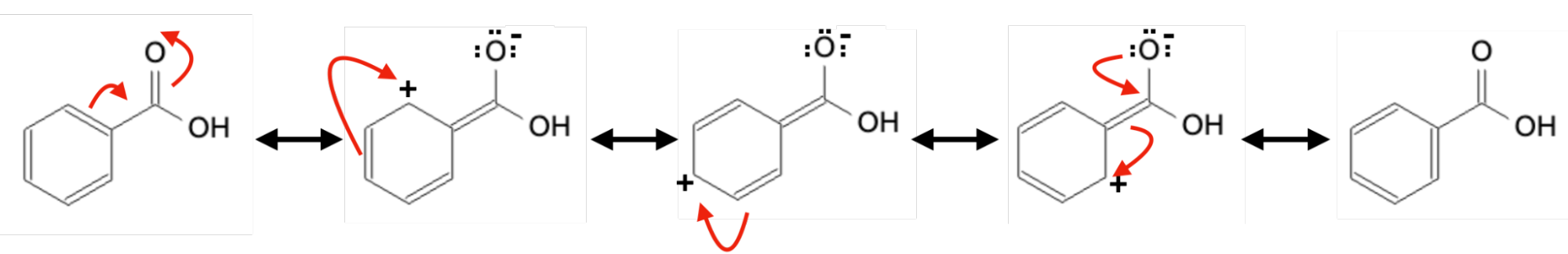Figure 2: Benzoic Acid Resonance

These 5 resonance structures allow benzoic acid to mix in organic/nonpolar solutions much more effectively than the 1 resonance structure for crotonic acid: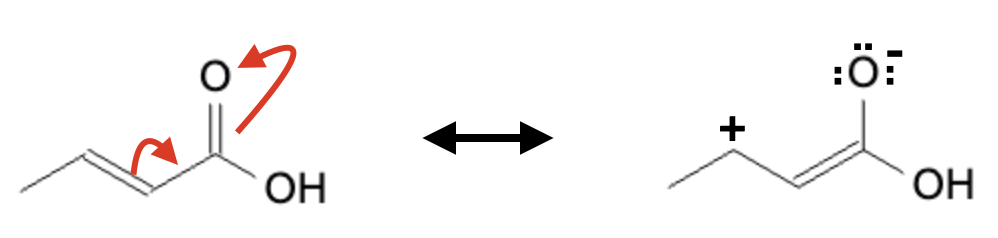Figure 3: Crotonic Acid Resonance

### Conclusion

The experiment demonstrated the concept of distribution coefficients, where the solubility of a solute in different phases affects its distribution between those phases. The obtained distribution coefficients indicated that while crotonic acid was soluble in both phases (K=0.786), benzoic acid was nearly nine times more soluble in the organic phase than in the aqueous phase (K=8.85). This supports the hypothesis that benzoic acid would be more soluble in the organic phase. The increased resonance structures of benzoic acid predict that it would be more miscible in a nonpolar environment compared to a polar one.

Post Lab Questions

1. A 400mL aqueous solution containing 40g of malononitrile. To isolate it, you perform a liquid-liquid extraction. The solubility of malononitrile is 20g/100mL in ether and 13.3g/100mL in H2O. How many grams of malononitrile can be isolated with 3 extractions using 100mL of ether each time? To calculate this, follow the equations below: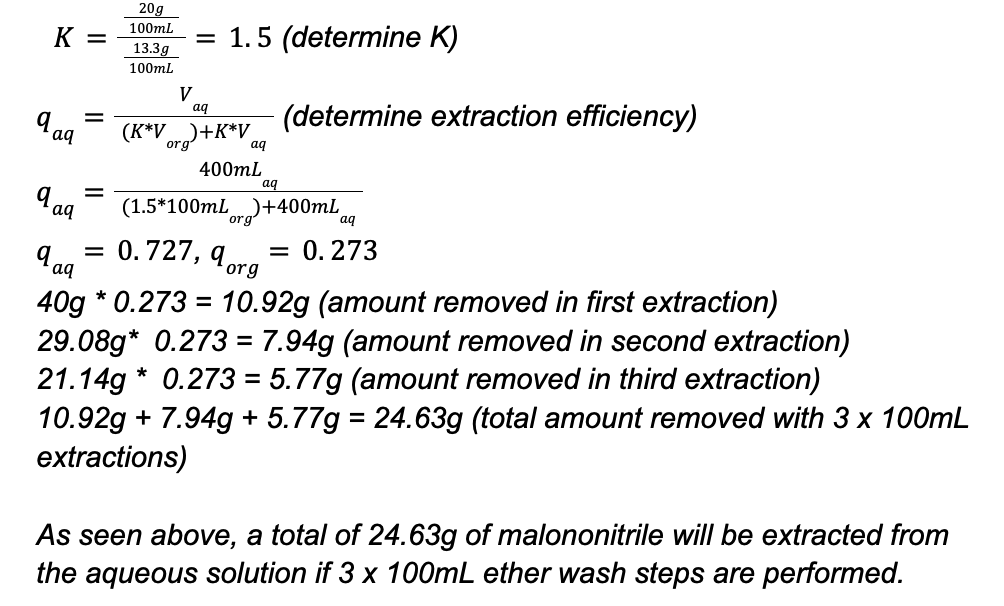As seen above, a total of 24.63g of malononitrile will be extracted from the aqueous solution if 3 x 100mL ether wash steps are performed.

1. How many grams can be extracted using a single 300mL extraction? To calculate this, follow the equations below: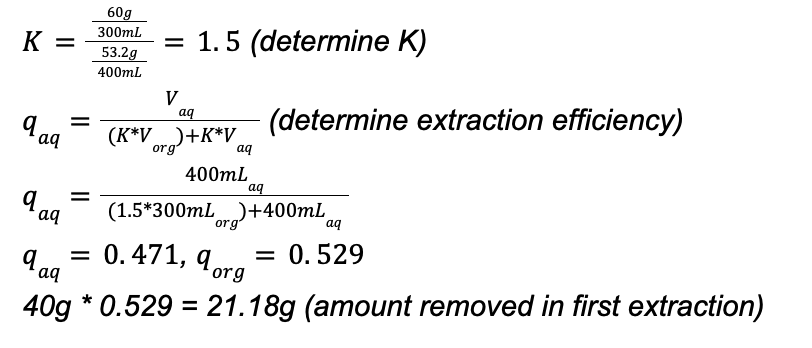As seen above, a total of 21.18g of malononitrile will be extracted from the aqueous solution if 1 x 300mL ether wash steps are performed.

1. A common mistake is removing the stopper from the separatory funnel during a separation; why is this a necessary step? Removal of the stopper is necessary to prevent the formation of a vacuum, which would prevent liquid from flowing out of the separatory funnel at a predictable, accurate rate.

2. The distribution coefficient (K) between hexanes and water for an unknown mixture is 7.5.

A) If a solution has 20.0g of this unknown in 100mL of H2O and extracts it with 100mL of hexanes, how many grams of unknown can be isolated?

B)If 4x25mL extractions are performed, how much would be removed?

C)How much mL of hexanes would it take to remove 98.5% with a single extraction?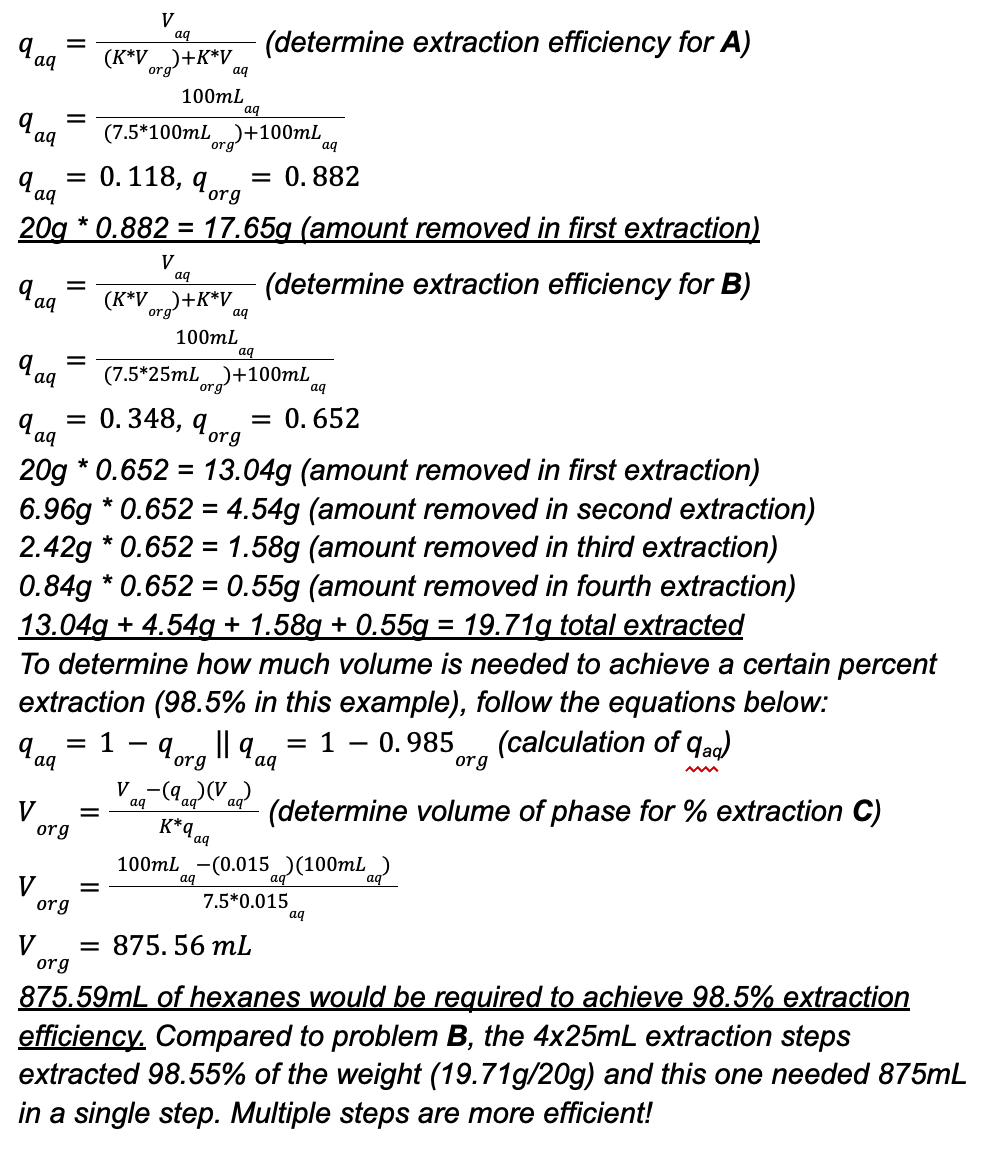Don't forget to check out our Molecule Namer study tool and our Reaction Solver study tool!